Скачать презентацию Physics of Magnetism Physical Principles of Magnetism

• Количество слайдов: 34Physics of Magnetism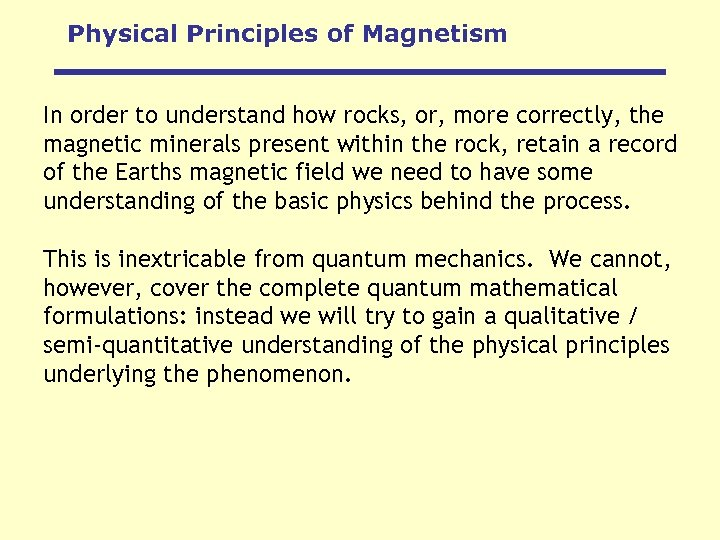Physical Principles of Magnetism In order to understand how rocks, or, more correctly, the magnetic minerals present within the rock, retain a record of the Earths magnetic field we need to have some understanding of the basic physics behind the process. This is inextricable from quantum mechanics. We cannot, however, cover the complete quantum mathematical formulations: instead we will try to gain a qualitative / semi-quantitative understanding of the physical principles underlying the phenomenon.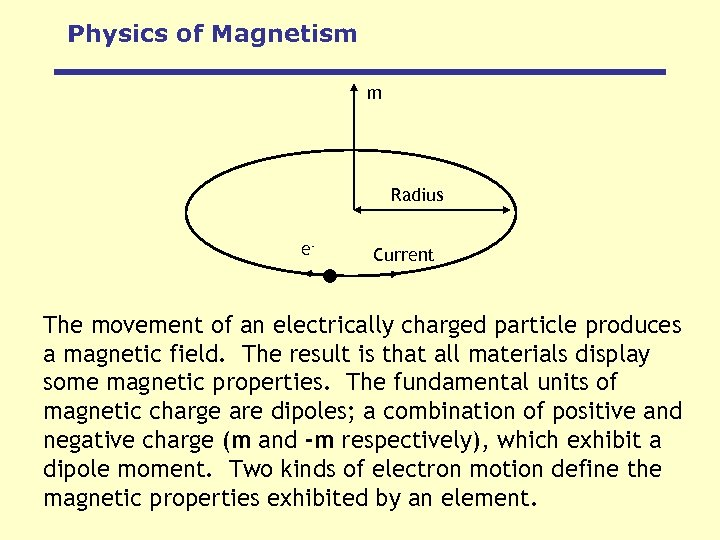Physics of Magnetism m Radius e- Current The movement of an electrically charged particle produces a magnetic field. The result is that all materials display some magnetic properties. The fundamental units of magnetic charge are dipoles; a combination of positive and negative charge (m and -m respectively), which exhibit a dipole moment. Two kinds of electron motion define the magnetic properties exhibited by an element.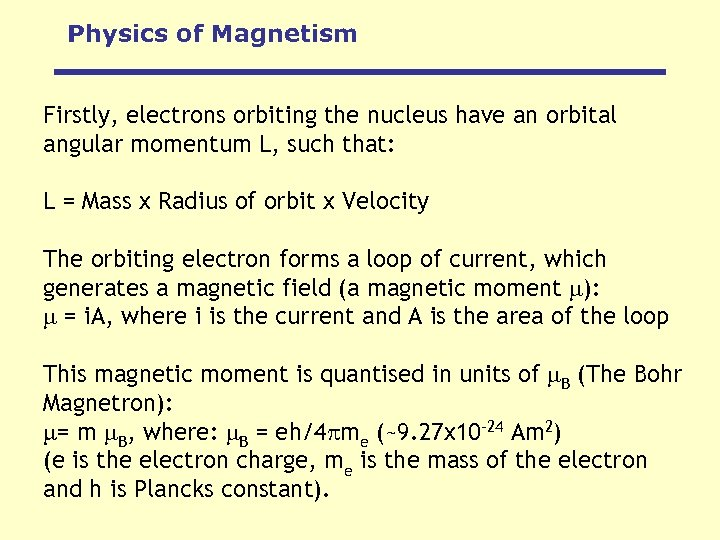Physics of Magnetism Firstly, electrons orbiting the nucleus have an orbital angular momentum L, such that: L = Mass x Radius of orbit x Velocity The orbiting electron forms a loop of current, which generates a magnetic field (a magnetic moment ): = i. A, where i is the current and A is the area of the loop This magnetic moment is quantised in units of B (The Bohr Magnetron): = m B, where: B = eh/4 me (~9. 27 x 10 -24 Am 2) (e is the electron charge, me is the mass of the electron and h is Plancks constant).Physics of Magnetism Secondly, the electron has a spin and a spin angular momentum S. Each electron spins around an axis, and that axis can have one of two possible orientations: either parallel or antiparallel to an external magnetic field. This spinning charge gives rise to a magnetic field: S = B The superposition of these forms of electron motion gives the total angular momentum of the atom. Magnetism in solids, however, is dominated by the magnetic moment associated with electron spin.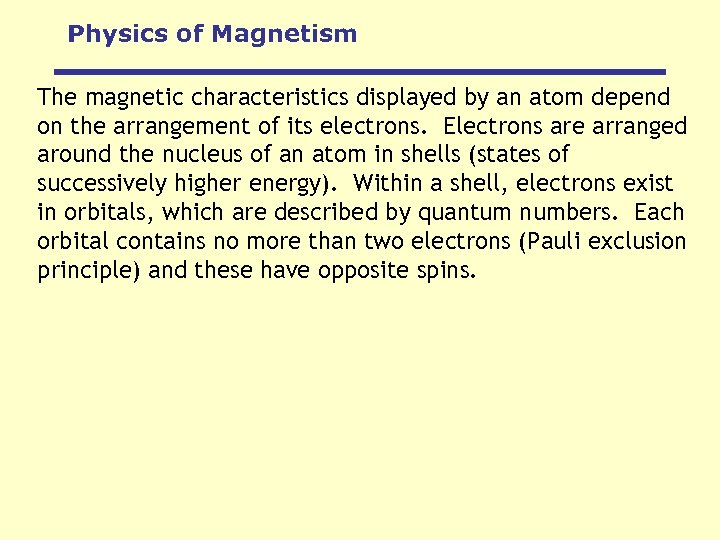Physics of Magnetism The magnetic characteristics displayed by an atom depend on the arrangement of its electrons. Electrons are arranged around the nucleus of an atom in shells (states of successively higher energy). Within a shell, electrons exist in orbitals, which are described by quantum numbers. Each orbital contains no more than two electrons (Pauli exclusion principle) and these have opposite spins.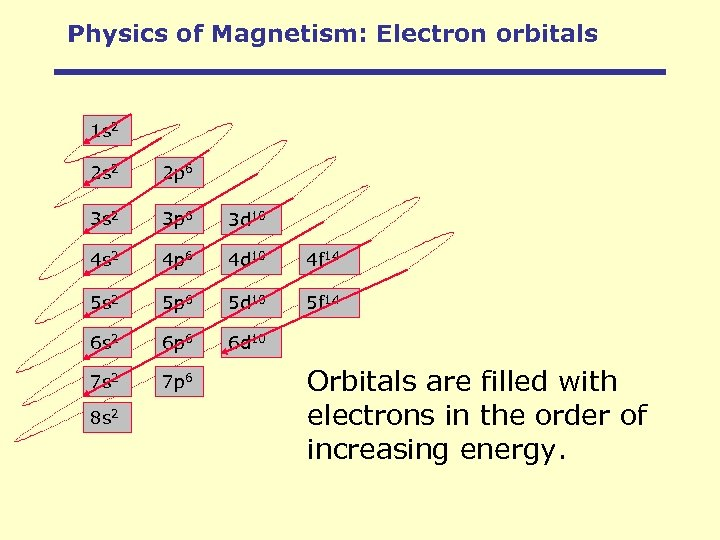Physics of Magnetism: Electron orbitals 1 s 2 2 p 6 3 s 2 3 p 6 3 d 10 4 s 2 4 p 6 4 d 10 4 f 14 5 s 2 5 p 6 5 d 10 5 f 14 6 s 2 6 p 6 6 d 10 7 s 2 7 p 6 8 s 2 Orbitals are filled with electrons in the order of increasing energy.Physics of Magnetism Electrons occupy specific energy levels, or orbitals, as they orbit the nucleus of an atom.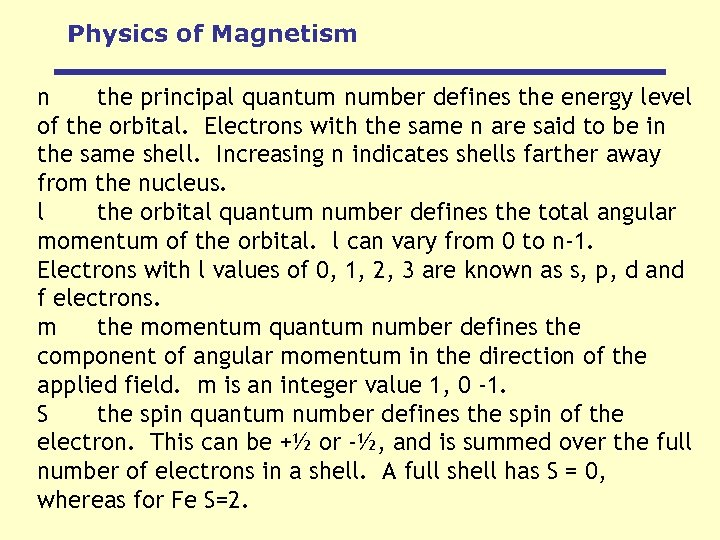Physics of Magnetism n the principal quantum number defines the energy level of the orbital. Electrons with the same n are said to be in the same shell. Increasing n indicates shells farther away from the nucleus. l the orbital quantum number defines the total angular momentum of the orbital. l can vary from 0 to n-1. Electrons with l values of 0, 1, 2, 3 are known as s, p, d and f electrons. m the momentum quantum number defines the component of angular momentum in the direction of the applied field. m is an integer value 1, 0 -1. S the spin quantum number defines the spin of the electron. This can be +½ or -½, and is summed over the full number of electrons in a shell. A full shell has S = 0, whereas for Fe S=2.Physics of Magnetism Atomic Number Element Na Mg K 1 s ↑↓ ↑↓ L 2 s ↑↓ ↑↓ 2 p 6 6 M 3 s ↑ ↑↓ 11 12 13 Al ↑↓ ↑↓ 6 ↑↓ ↑ 14 15 16 17 18 19 20 Si P S Cl Ar K Ca ↑↓ ↑↓ ↑↓ ↑↓ 6 6 6 6 ↑↓ ↑↓ ↑ ↑ ↑↓ ↑↓ ↑↓ N 4 s 3 p ↑ ↑↓ ↑↓ ↑ ↑ ↑ ↑↓ ↑↓ ↑↓ ↑ ↑↓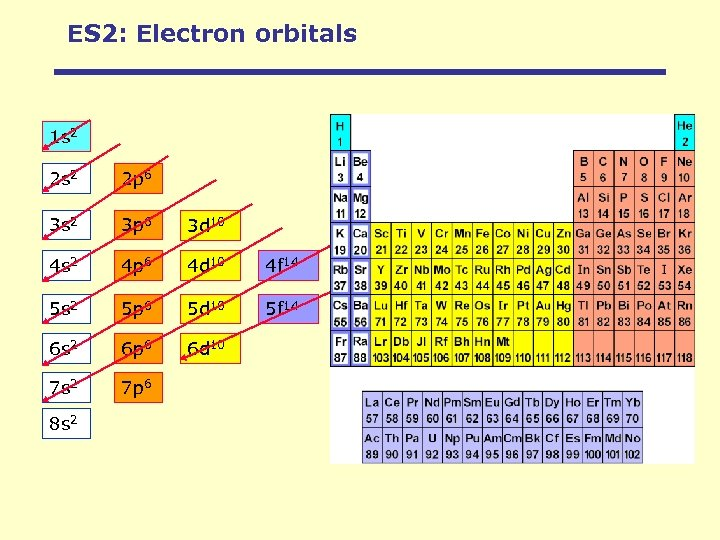ES 2: Electron orbitals 1 s 2 2 p 6 3 s 2 3 p 6 3 d 10 4 s 2 4 p 6 4 d 10 4 f 14 5 s 2 5 p 6 5 d 10 5 f 14 6 s 2 6 p 6 6 d 10 7 s 2 7 p 6 8 s 2Physics of Magnetism Atomic Number Element 21 22 Inner Shells Sc Ti 3 d ↑ ↑ ↑ 4 s ↑↓ ↑↓ 23 V ↑ ↑↓ 24 25 26 27 28 29 30 Cr Mn Fe Co Ni Cu Zn ↑ ↑ ↑↓ ↑ ↑ ↑↓ ↑↓ ↑↓ ↑ ↑ ↑↓ ↑↓ ↑↓ ↑↓ 1 s 22 p 63 s 23 p 6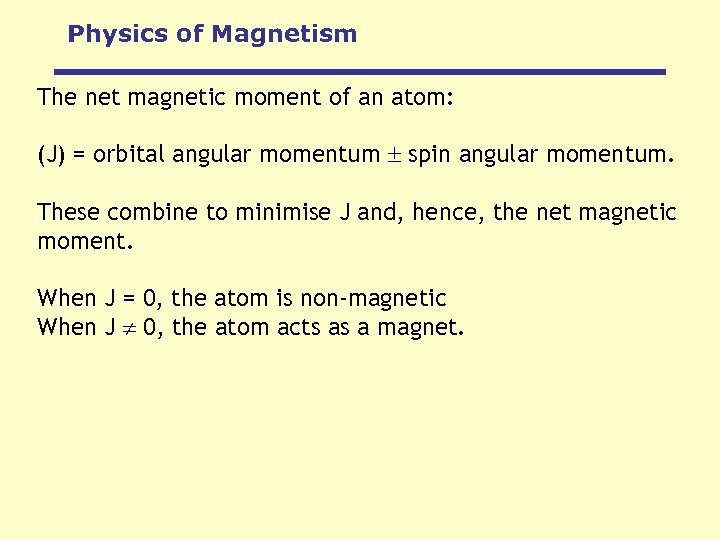Physics of Magnetism The net magnetic moment of an atom: (J) = orbital angular momentum spin angular momentum. These combine to minimise J and, hence, the net magnetic moment. When J = 0, the atom is non-magnetic When J 0, the atom acts as a magnet.Physics of Magnetism As the magnetic moment in solids is dominated by the magnetic moment associated with electron spin, an atom will possess an overall magnetic moment where there are unpaired electrons in an orbital (i. e. the spin moments are not cancelled). As single electrons are added the spin moments are combined, and the resultant magnetic moment (from spin) is at a maximum when the outer shell is half-full, and decreases as further electrons are added to the outer shell until it is full.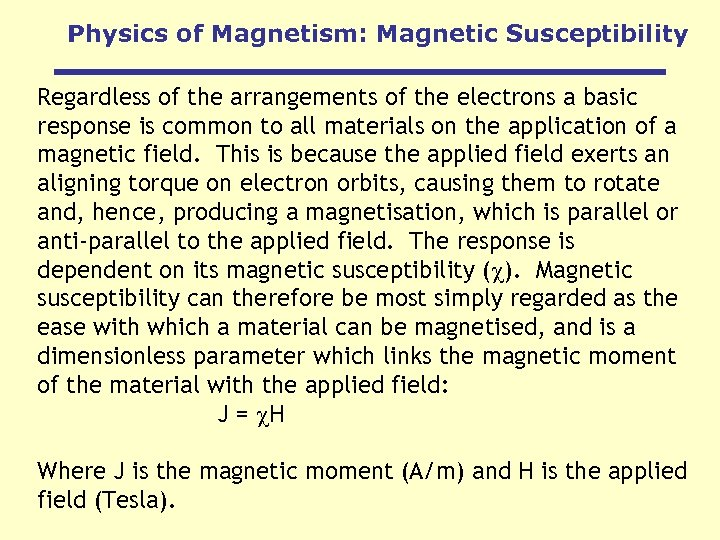Physics of Magnetism: Magnetic Susceptibility Regardless of the arrangements of the electrons a basic response is common to all materials on the application of a magnetic field. This is because the applied field exerts an aligning torque on electron orbits, causing them to rotate and, hence, producing a magnetisation, which is parallel or anti-parallel to the applied field. The response is dependent on its magnetic susceptibility ( ). Magnetic susceptibility can therefore be most simply regarded as the ease with which a material can be magnetised, and is a dimensionless parameter which links the magnetic moment of the material with the applied field: J = H Where J is the magnetic moment (A/m) and H is the applied field (Tesla).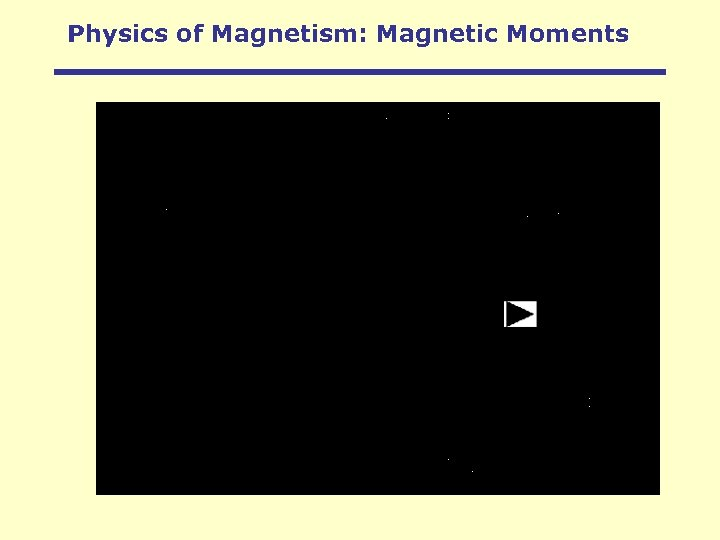Physics of Magnetism: Magnetic Moments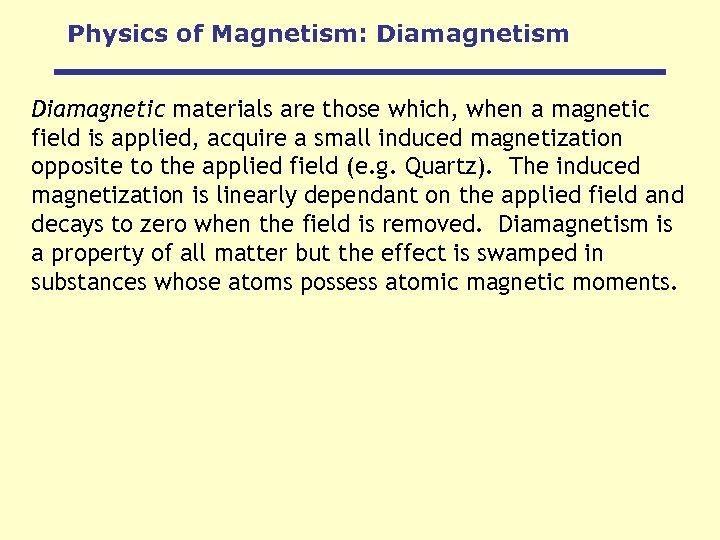Physics of Magnetism: Diamagnetism Diamagnetic materials are those which, when a magnetic field is applied, acquire a small induced magnetization opposite to the applied field (e. g. Quartz). The induced magnetization is linearly dependant on the applied field and decays to zero when the field is removed. Diamagnetism is a property of all matter but the effect is swamped in substances whose atoms possess atomic magnetic moments.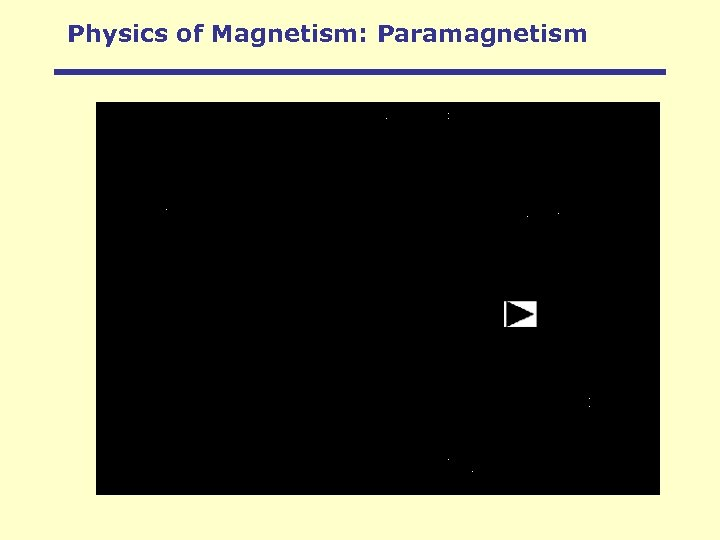Physics of Magnetism: Paramagnetism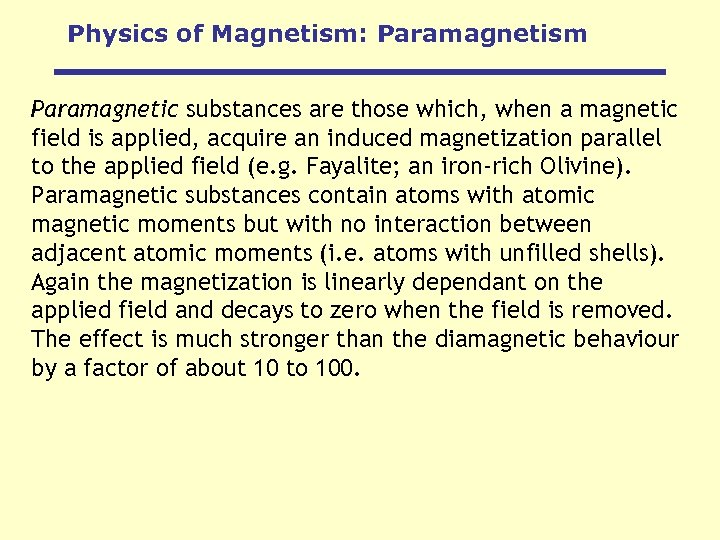Physics of Magnetism: Paramagnetism Paramagnetic substances are those which, when a magnetic field is applied, acquire an induced magnetization parallel to the applied field (e. g. Fayalite; an iron-rich Olivine). Paramagnetic substances contain atoms with atomic magnetic moments but with no interaction between adjacent atomic moments (i. e. atoms with unfilled shells). Again the magnetization is linearly dependant on the applied field and decays to zero when the field is removed. The effect is much stronger than the diamagnetic behaviour by a factor of about 10 to 100.Physics of Magnetism: Permanent magnetism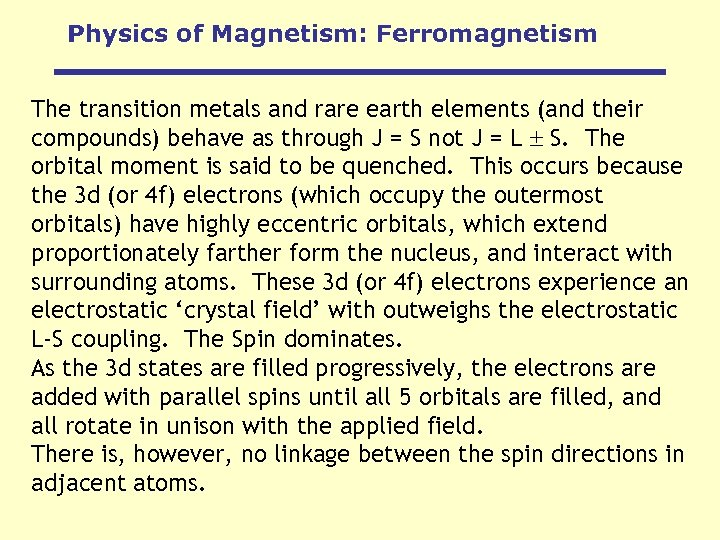Physics of Magnetism: Ferromagnetism The transition metals and rare earth elements (and their compounds) behave as through J = S not J = L S. The orbital moment is said to be quenched. This occurs because the 3 d (or 4 f) electrons (which occupy the outermost orbitals) have highly eccentric orbitals, which extend proportionately farther form the nucleus, and interact with surrounding atoms. These 3 d (or 4 f) electrons experience an electrostatic ‘crystal field’ with outweighs the electrostatic L-S coupling. The Spin dominates. As the 3 d states are filled progressively, the electrons are added with parallel spins until all 5 orbitals are filled, and all rotate in unison with the applied field. There is, however, no linkage between the spin directions in adjacent atoms.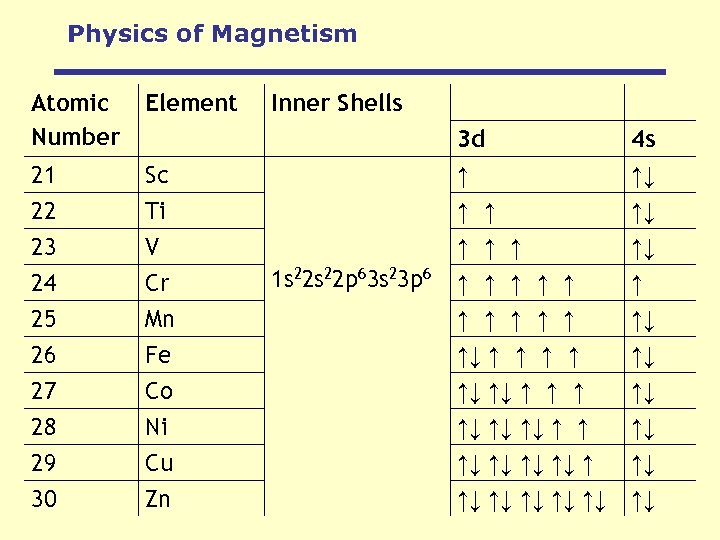Physics of Magnetism Atomic Number Element 21 22 Inner Shells Sc Ti 3 d ↑ ↑ ↑ 4 s ↑↓ ↑↓ 23 V ↑ ↑↓ 24 25 26 27 28 29 30 Cr Mn Fe Co Ni Cu Zn ↑ ↑ ↑↓ ↑ ↑ ↑↓ ↑↓ ↑↓ ↑ ↑ ↑↓ ↑↓ ↑↓ ↑↓ 1 s 22 p 63 s 23 p 6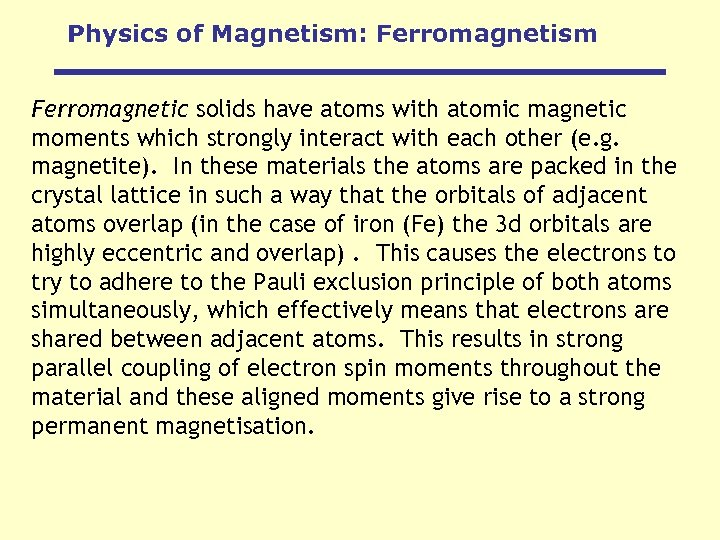Physics of Magnetism: Ferromagnetism Ferromagnetic solids have atoms with atomic magnetic moments which strongly interact with each other (e. g. magnetite). In these materials the atoms are packed in the crystal lattice in such a way that the orbitals of adjacent atoms overlap (in the case of iron (Fe) the 3 d orbitals are highly eccentric and overlap). This causes the electrons to try to adhere to the Pauli exclusion principle of both atoms simultaneously, which effectively means that electrons are shared between adjacent atoms. This results in strong parallel coupling of electron spin moments throughout the material and these aligned moments give rise to a strong permanent magnetisation.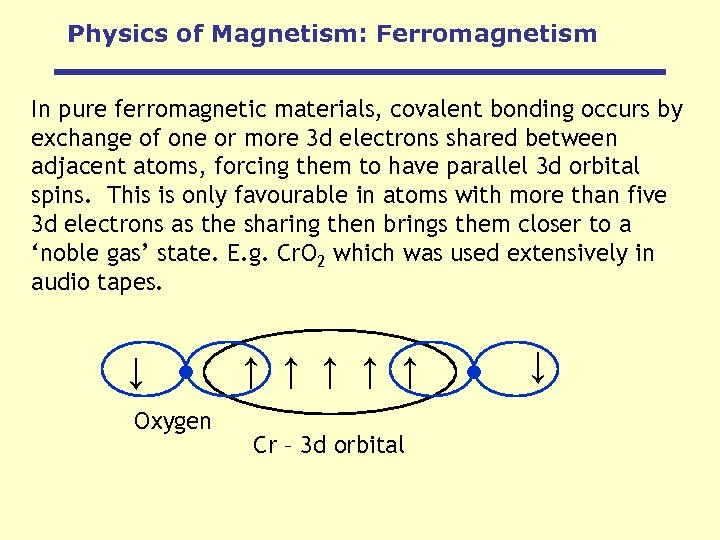Physics of Magnetism: Ferromagnetism In pure ferromagnetic materials, covalent bonding occurs by exchange of one or more 3 d electrons shared between adjacent atoms, forcing them to have parallel 3 d orbital spins. This is only favourable in atoms with more than five 3 d electrons as the sharing then brings them closer to a ‘noble gas’ state. E. g. Cr. O 2 which was used extensively in audio tapes. Cr – 3 d orbital ↑ ↑ Oxygen ↑ ↑ ↑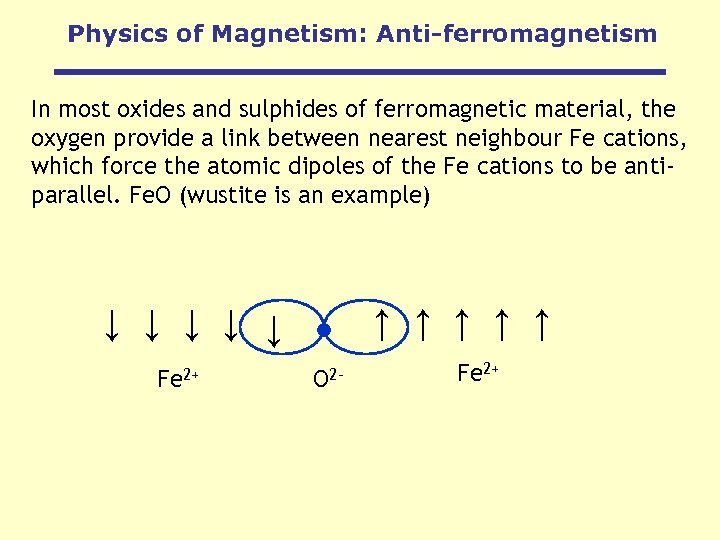Physics of Magnetism: Anti-ferromagnetism In most oxides and sulphides of ferromagnetic material, the oxygen provide a link between nearest neighbour Fe cations, which force the atomic dipoles of the Fe cations to be antiparallel. Fe. O (wustite is an example) ↑ ↑ ↑ ↑ ↑ Fe 2+ O 2 - Fe 2+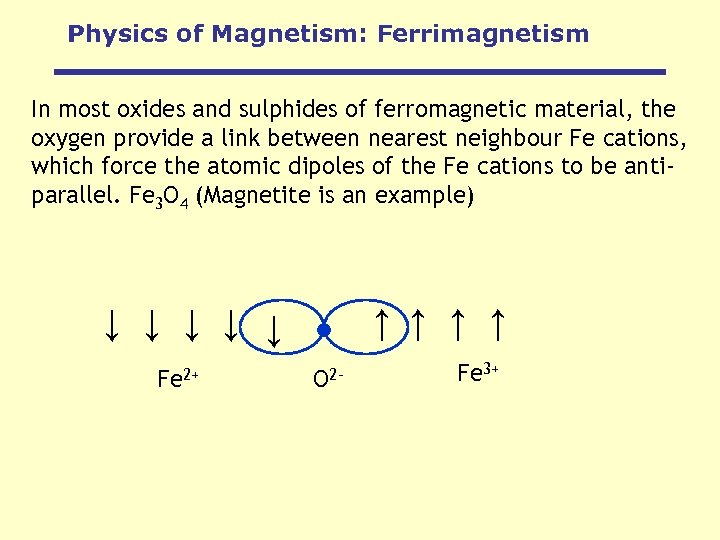Physics of Magnetism: Ferrimagnetism In most oxides and sulphides of ferromagnetic material, the oxygen provide a link between nearest neighbour Fe cations, which force the atomic dipoles of the Fe cations to be antiparallel. Fe 3 O 4 (Magnetite is an example) ↑ ↑ ↑ ↑ ↑ Fe 2+ O 2 - Fe 3+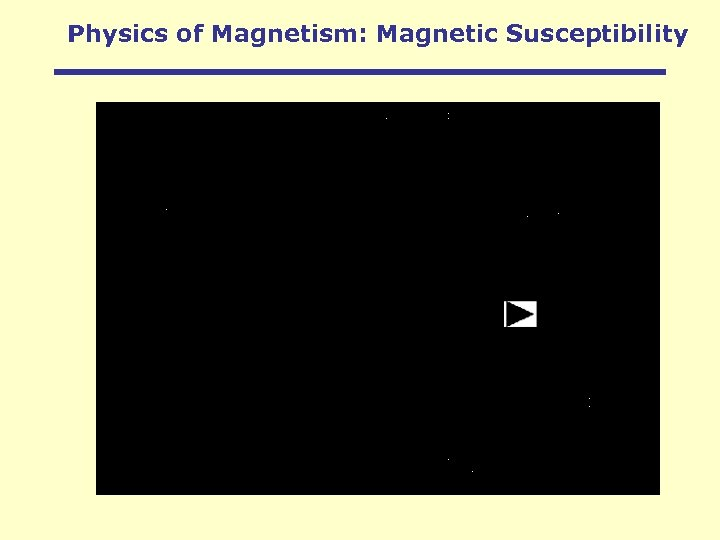Physics of Magnetism: Magnetic Susceptibility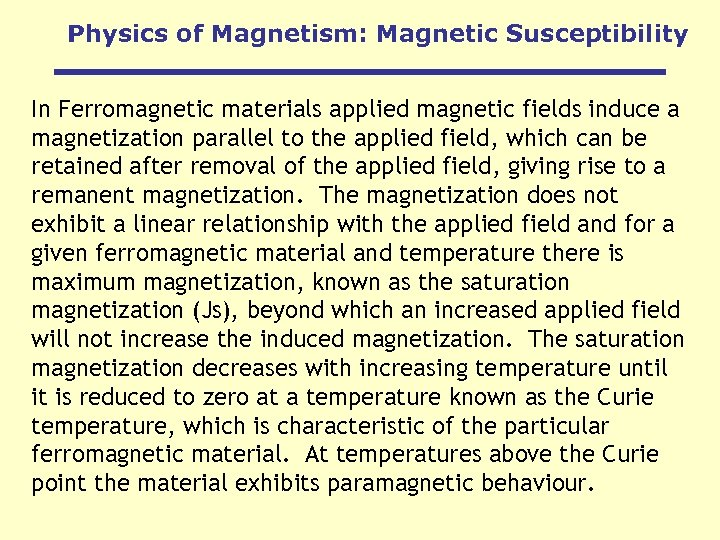Physics of Magnetism: Magnetic Susceptibility In Ferromagnetic materials applied magnetic fields induce a magnetization parallel to the applied field, which can be retained after removal of the applied field, giving rise to a remanent magnetization. The magnetization does not exhibit a linear relationship with the applied field and for a given ferromagnetic material and temperature there is maximum magnetization, known as the saturation magnetization (Js), beyond which an increased applied field will not increase the induced magnetization. The saturation magnetization decreases with increasing temperature until it is reduced to zero at a temperature known as the Curie temperature, which is characteristic of the particular ferromagnetic material. At temperatures above the Curie point the material exhibits paramagnetic behaviour.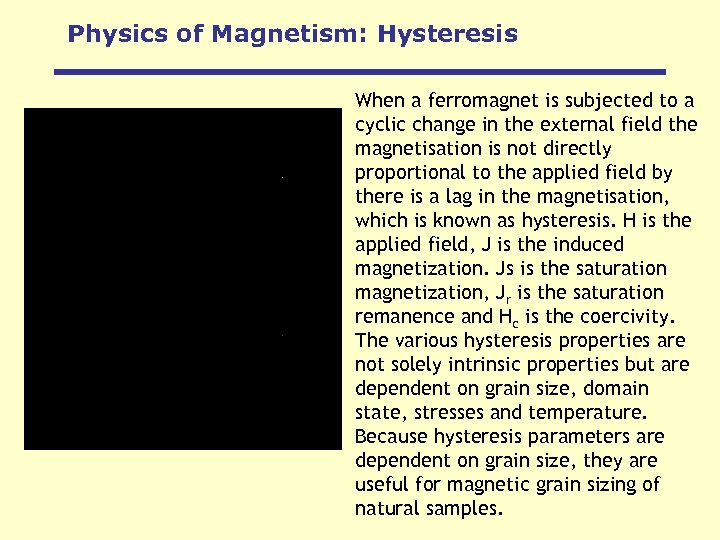Physics of Magnetism: Hysteresis When a ferromagnet is subjected to a cyclic change in the external field the magnetisation is not directly proportional to the applied field by there is a lag in the magnetisation, which is known as hysteresis. H is the applied field, J is the induced magnetization. Js is the saturation magnetization, Jr is the saturation remanence and Hc is the coercivity. The various hysteresis properties are not solely intrinsic properties but are dependent on grain size, domain state, stresses and temperature. Because hysteresis parameters are dependent on grain size, they are useful for magnetic grain sizing of natural samples.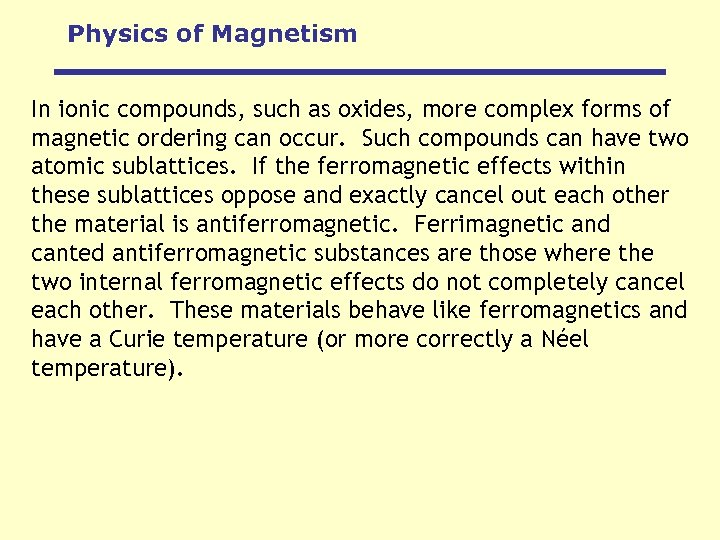Physics of Magnetism In ionic compounds, such as oxides, more complex forms of magnetic ordering can occur. Such compounds can have two atomic sublattices. If the ferromagnetic effects within these sublattices oppose and exactly cancel out each other the material is antiferromagnetic. Ferrimagnetic and canted antiferromagnetic substances are those where the two internal ferromagnetic effects do not completely cancel each other. These materials behave like ferromagnetics and have a Curie temperature (or more correctly a Néel temperature).Physics of Magnetism: Temperature Effects 0°K < Tc T > Tc Alignment of magnetic moments at various temperatures: at 0°K there is perfect alignment, but above this the spin moments precess about the average direction due to thermal activation. Above the Curie temperature they are random.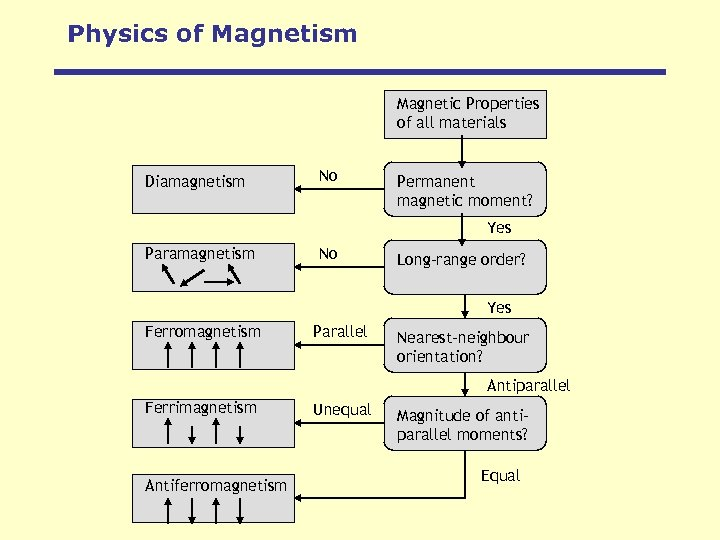Physics of Magnetism Magnetic Properties of all materials Diamagnetism No Permanent magnetic moment? Yes Paramagnetism No Long-range order? Yes Ferromagnetism Parallel Nearest-neighbour orientation? Antiparallel Ferrimagnetism Antiferromagnetism Unequal Magnitude of antiparallel moments? Equal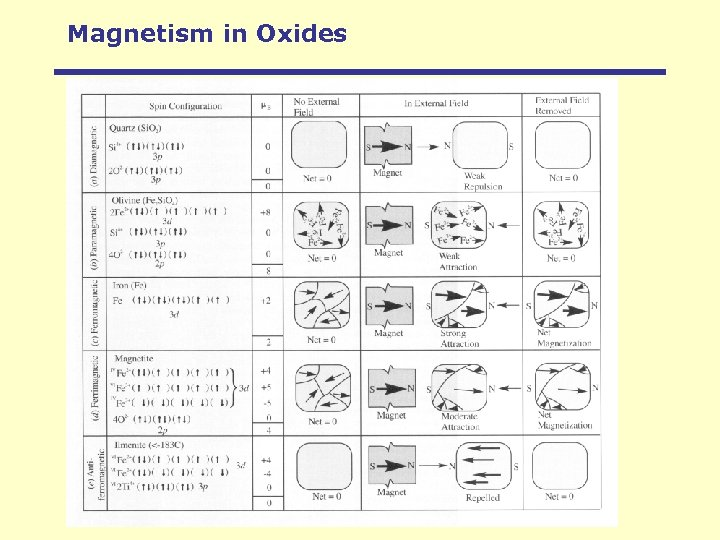Magnetism in OxidesMagnetism in Oxides Ilmeno. Haematite series Titano. Magnetite series